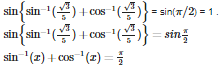# Inverse Trigonometric Functions - Online Test

Q1. If is equal to
Explaination / Solution: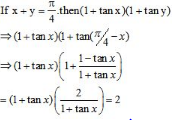Q2. The period of the function f(x)=cos 4x + tan 3x is
Explaination / Solution:

f(π)=(cos4π+tan3π)gives the same value as f (0) .Therefore,the period of the function is π

Q3. The general solution of the equation cotθ−tanθ=secθis(n∈I)
Explaination / Solution: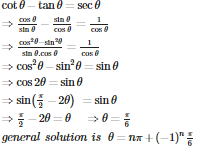Q4. is
Explaination / Solution:

Q5. The value ofis
Explaination / Solution: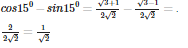Q6. he value of is
Explaination / Solution: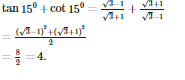Q7.  is
Explaination / Solution:

Because both  lies in 3rd quadrant. In 3rd quadrant values of both  sine and cosine functions are negative.

Q8. If sin A + cos A = 1, then sin 2A is equal to
Explaination / Solution:

1 = 1 + Sin 2A

so, Sin 2A = 0

Hence A = 0

Q9. The value of  is
Explaination / Solution:

The value of  =

Q10.  is equal to
Explaination / Solution: